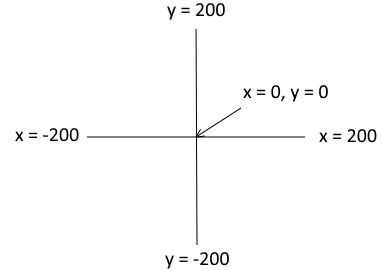# 12.6. Turtles And Randomness¶

We can use random numbers to make the results of turtle drawings unpredictable. The program below will pick a random number between 50 and 199 and call it squareSize. That number gets passed to the drawSquare function as the size of the square to draw. Each time you run the program, the square should be a different size.

As a reminder, here are the turtle commands: Turtle procedures

Note that although we want the size of the square to be random, it is important that all the sides of the square are the same size. That is why we pick the random number in the main part of the program. If we picked a random number on line 3 as we draw each side, each side would be a different length! Try changing line 3 to read turtleName.forward(random.randrange(50, 200)) to see for yourself.

We can also pick a random location for the turtle to start in. Remember that the turtle uses this coordinate system:The coordinates for the drawing space. Note that the center is x = 0 and y = 0.¶

Because the square procedure is going to make a square up to 200 wide and tall extending to the right and down from where we start, I don’t want it to start to the right or below the point 0, 0 or it might go off screen. I am fine with it starting to the left of or above that location, but I don’t want it to start right at the edge of the screen. So I want the starting x location to be between -190 and 0 and the starting y to be between 0 and 190. So this version of the program uses goto(x, y) and random numbers to pick a random starting location before drawing the square.

Try the program a few times. Each time you should get different squares.

Now let’s make it a little more interesting by picking a random color each time the program runs. We have added a procedure called changeColor. If you pass it the name of a turtle, and three numbers between 0-255 representing red, green, and blue values, it will make a hexadecimal color code that represents the color with that much red/green/blue. It will then use that to set the color of the turtle. Your task is to write code to pick three random numbers to use for the color values.

In the location marked TODO (line 38), add lines of code to make three random values that are each 0-255 and name them red, green, and blue.